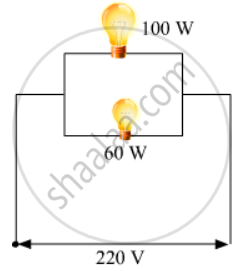# Two Lamps, One Rated 100 W at 220 V, and the Other 60 W at 220 V, Are Connected in Parallel to Electric Mains Supply. What Current is Drawn from the Line If the Supply Voltage is 220 V? - Science

Two lamps, one rated 100 W at 220 V, and the other 60 W at 220 V, are connected in parallel to electric mains supply. What current is drawn from the line if the supply voltage is 220 V?

Two lamps, one rated 100 W; 220 V, and the other 60 W; 220 V, are connected in parallel to electric mains supply. Find the current drawn by two bulbs from the line, if the supply voltage is 220 V.

#### Solution 1

Both the bulbs are connected in parallel. Therefore, potential difference across each of them will be 220 V, because no division of voltage occurs in a parallel circuit.
Current drawn by the bulb of rating 100 W is given by,Power = Voltage x Current
Current =  Power/Voltage = 60/220 A
Hence, current drawn from the line = 100/220 + 60/220 = 0.727 A

#### Solution 2For first bulb:

P = 100 W, V = 220V

As we know, P = v^2/R

Thus, resistance of first bulb, R_1 = v^2/P = 220^2/100 = 484 Ω

For second bulb:

Thus, resistance of second bulb, R_2 = v^2/P = 220^2/60 = 806.67 Ω

Since, the two bulbs are connected in parallel, therefore

Total resistance = (R_1R_2)/(R_1 + R_2) = (484xx806.67)/(484+806.67) = 302.5 Ω

Hence, the current drawn by two bulbs is

I = 220/302.5 = 0.73 A

Concept: Electrical Power
Is there an error in this question or solution?